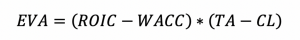# The EVA Firm Valuation Model [Economic Value Added][FCFF Inputs]

In a different article, we introduced the FCFF valuation model. Here we will tie in the FCFF model with EVA (economic value added), a financial metric that estimates a company’s profit through the net surplus created from its investments.

Mathematically, it can be expressed as (Profit of the firm) – (Cost of capital of the firm).

Or:

The difference of invested capital (ROIC) minus the weighted average cost of capital (WACC) multiplied by the difference of total assets minus current liabilities.Economic value added is a valuation framework that is basically the same in purpose as an FCFF model.

Accordingly, inputs from the FCFF model work to form our EVA valuation.

We can determine our EVA figure by using EBIT(1-t) as a measure of the profit of the firm and subtracting WACC (CI) (weighted average cost of capital of capital invested), which designates the firm’s cost of capital.

EBIT(1-t) represents Earnings Before Interest and Taxes multiplied by one minus the tax rate to ensure that taxes are already taken into account.

WACC (CI), as noted, means the Weighted Average Cost of Capital of Capital Invested, which measures a company’s ability to finance new projects.

The lower the WACC, the better, meaning the firm can finance these projects more easily.

For example, a company with a cost of capital of three percent can more easily find profitable investments relative to a company with a hurdle rate of ten percent.

We will go through each component of the spreadsheet individually:

### 1. EBIT (1-t)

Equal to (Revenues) – (Operating Expenses), with that figure multiplied by 1 – (Tax rate).

The EBIT (1-t) figure is already set up in the FCFF Valuation spreadsheet. So in order to import this value to the EVA Valuation spreadsheet we can use single-hash quotes naming the spreadsheet we want to import from, followed by an exclamation point, then the name of the cell where the desired figure is located.

For example, our EBIT (1-t) figure from the base period is already calculated on our FCFF Valuation spreadsheet in cell B42.

So we write: =’FCFF Valuation’!B42

And our value from that cell will appear in our EVA sheet.

### 2. WACC (CI)

Next we must subtract WACC (CI) to obtain our EVA figure.

We won’t go into the complete definition of WACC all the way to the level of the basic inputs because then the calculation will become very messy with something like two dozen numbers to consider.

That’s why feeding inputs in from the FCFF spreadsheet becomes so valuable in the time that it saves and consequently avoids an inconvenient computational mess in terms of having to design all new formulas.

I will keep WACC (CI) in terms of seven variables:

• (Cost of equity * Proportion of equity + After-tax cost of debt * Cost of capital) * (Initial capital invested in the firm + Net capital expenditures + Change in working capital)

### 3. EVA

EVA, or economic value added, is the result of subtracting WACC (CI) from EBIT (1-t).

### 4. Terminal EVA

Since we are attempting to estimate value over the life of the firm and not merely within the time period established on the spreadsheet, we need to calculate a terminal value using a perpetuity growth model.

In our FCFF valuation model, we broke our firm into three stages of growth: five years of high growth, a five-year transition period from high growth into stable growth, and a stable growth period that’s assumed to last indefinitely.

Terminal EVA is done by taking the difference between the terminal year EBIT(1-t) and WACC (CI) figures and then taking that value and dividing it by cost of capital once the stable growth period begins minus the revenue growth rate in the final period.

Expressed mathematically, it appears as:

• [ (Terminal value of EBIT(1-t) – Terminal value of WACC (CI)) / (Cost of capital in the stable growth period – Revenue growth rate in the stable growth period) ]

### 5. Present Value

The present value of a company within a given year is given by the EVA and Cumulative WACC in the same time period.

For present value in the final year going forward, it is equal to (EVA in the final year + Terminal EVA) / (Cumulative WACC in the final year).

At this point, with those variables settled, we can begin the three-factor calculation that goes into determining firm value.

### 6. Present Value of EVA

This is the sum of all present values calculated.

### 7. Capital Invested

The initial sum of capital invested in the firm when starting out. Will be added to the present value of EVA.

### 8. Present Value of Change in Capital of Final Year

Our model encompasses ten years, so our “final year” as stipulated in the variable name is the tenth period.

To calculate we take the “Terminal Year Capital Invested” and subtract the “Ending Capital Invested” and take this figure and divide by “Cumulated WACC”, which is pulled right off our FCFF spreadsheet.

This value is made to balance assumptions regarding capital invested, return on capital, and stable growth.

### 9. Firm Value

Firm value is the sum of the aforementioned three factors.

It will give us the same firm value as determined in our FCFF spreadsheet.

## Conclusion

The focus of this EVA model is to reconcile valuation directly from the FCFF approach.

Both are business valuation approaches within the discounted cash flow model analysis framework.

We achieved the same business valuation assessment, but through a different calculation metric in the form of economic value added.

In the end, while they spit out the same valuation figure, different methodological techniques are used to derive this final output.

by